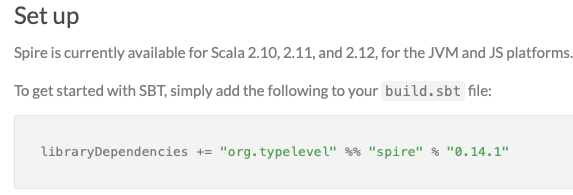# Converting code using spire.Rational from 2.12 to 2.13

I have code using the `Rational` class from `spire.math` in a scala 2.12 project.
I’d like to convert this project to scala 2.13. But it seems spire has not been ported to 2.13.
Is that correct?
Which `Rational` number library should I use in 2.13?

Can I use the `Fractional` trait instead?
The code is not really complicated, so I don’t mind refactoring it a bit.

``````  def rationalFoldTest(bounds:List[Int],randomize:Boolean):Unit = {
import spire.implicits._
import spire.math._
import treereduce.TreeParallelReduce._
import treereduce.TreeReduce._
import treereduce.TreeReducible._
import gnuplot.GnuPlot.gnuPlot
def rationalAdd(a: Rational, b: Rational): Rational = a + b

val zero = Rational(0, 1)
val rawDataForPlot = (for {r <- bounds.sorted.reverse.par // allow computation in parallel
piercedInterval = (if (randomize)
scala.util.Random.shuffle( (( -r to -1) ++ (1 to r)).toList)
else
(( -r to -1) ++ (1 to r)).toList)

t1 = time(3, s"\$r  treeMapReduce", {
val sum = piercedInterval.treeMapReduce(Rational(0, 1))(Rational(1, _), _ + _)
assert(sum === zero)
})
t2 = time(3, s"\$r  pairMapReduce", {
val sum = pairMapReduce(piercedInterval)(Rational(0, 1), Rational(1, _), rationalAdd) //(indexedSeqPairable)
assert(sum === zero)
})
t3 = time(3, s"\$r           fold", {
val sum = piercedInterval.map {
Rational(1, _)
}.foldLeft(Rational(0, 1))(_ + _)
assert(sum === zero)
})
_ = println()
} yield (r.toDouble, t1, t2, t3)).toList.sortBy(_._1)

val rs = rawDataForPlot.map(_._1)
val t1s = rawDataForPlot.map(_._2)
val t2s = rawDataForPlot.map(_._3)
val t3s = rawDataForPlot.map(_._4)
val dataForPlot = List(("tree-fold", rs, t1s)
, ("pair-wise-fold", rs, t2s)
, ("fold-left", rs, t3s))
gnuPlot(dataForPlot)(
title = "Fold Strategy Performance of Rational Addition" + (if (randomize) " (shuffled)" else " (sorted)"),
comment = "Fold Strategy Performance of Rational Addition",
xAxisLabel = "Number of terms (density)", xLog = true,
yAxisLabel = "Time (ms)", yLog = true,
grid = true,
outputFileBaseName = "rational-addition" + (if (randomize) "-random" else ""))
}
``````

Thanks, but the spire website says it is not supported for 2.13.What does “0.17.0-RC1” mean? I have updated my `build.sbt` to use the version you recommend, and it does in fact seem to work.

Really it doesn’t. What’s going on here is that it’s not officially released for 2.13 yet; that’s quite different from “not supported”. It looks to me like they just haven’t had a lot of free time to harden support for 2.13, so it’s going slowly.

“RC1” is the more or less universal abbreviation for “release candidate 1”. This means that they are in the process of releasing it, but not promising that it is bug-free, and usually implies that they would like people to use it and report any problems found. This release candidate is only a couple of weeks old, so it looks to me like they are actively working on it.

2 Likes